StatLect

# Size of a test

In hypothesis testing, the size of a test is the (maximum) probability of committing a Type I error, that is, of incorrectly rejecting the null hypothesis when it is true.## Null hypothesis

Suppose that we are conducting a test about a parameterthat can take any value in a parameter space.

The null hypothesisis thatbelongs to a given set:## Power function

Denote bythe power function of the test.

For any, the functiongives us the probability of rejecting the null hypothesis when the true parameter is: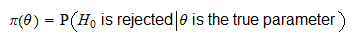Note thatdepends on:

• the true parameter;

• the null hypothesisthat we are testing;

• the statistical procedure (test statistic and critical region) used to decide whether to reject the null.

## Definition

The size of the test can be defined as follows.

Definition The size of the test, denoted by, isThus, we consider all the cases in which the null is true ().

For each case, we compute the probability of (incorrect) rejection.

The size is equal to the largest value we find (worst-case scenario).

## Simple vs composite hypothesis

When the null hypothesis is simple, that is, the setcontains only one parameter (denote it by), the above definition becomesThus, we have two cases:

1. if the null hypothesis does not specify an exact value for the parameter, but a whole set of parameters, then we need to take the maximum of the power function over that set in order to compute the size;

2. otherwise, if the null hypothesis specifies only one parameter, then it suffices to compute the value of the power function corresponding to that parameter.

## Size vs level of significance

Many authors use the term level of significance as a synonym for size.

For many others, the level of significance is an upper bound to the size, that is, a constantthat satisfies## Example - Size of a test for the mean

For example, suppose that we are testing the null hypothesis that the meanof a normal distribution is equal to.

The variance of the distribution, denoted by, is supposed to be known.

### How the test is conducted

We observe a sample ofindependent drawsfrom the distribution and we compute the z-statistic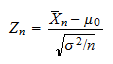whereis the sample mean: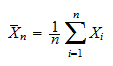We select a critical valueand reject the null hypothesis if### How the size is derived

It can be proved (see Hypothesis testing about the mean) that the power function of the test is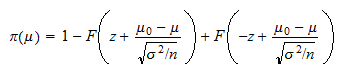whereis the cumulative distribution function of a standard normal random variable.

The size of the test is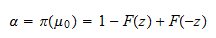By the symmetry of the standard normal distribution around 0, we have that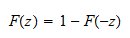As a consequence, we can write the size of the test as### How to visualize the size

In order to better understand this result, consider that under the null hypothesis the z-statistic has a standard normal distribution, that is, a normal distribution with mean equal toand variance equal to.

If you set, for example,, then you will reject the null in two cases:

• if the value of the z-statistic is less than, that is, if;

• if the value of the z-statistic is more than, that is, if.

But we know that ifhas a standard normal distribution, then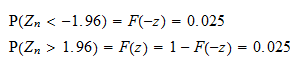Thus the size of the test is 5%: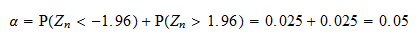The following plot shows the probability density function of the z-statistic.The black vertical segments indicate the two critical values.

When the value of the z-statistic falls in one of the two tails of the distribution (which are separated from the center of the distribution by the two critical values), the null hypothesis is incorrectly rejected.

The area under the probability density function in the two tails, colored with turquoise, is the probability of rejection, that is, the size of the test.

The area under the probability density function in the center of the distribution, colored with lavender, is the probability of acceptance.

## How to adjust the size of a test

In the previous example the size of the test was 5%. What if we want to decrease the size to 1%? How can this be achieved?

In general, the size of a test can be modified by changing the critical value(s) of the test, that is, by reducing or increasing the size (and hence the probability) of the critical region (remember that the critical region is the set of values of the test statistic that lead to rejection of the null hypothesis).

In the previous example, we can decrease the size of the test by increasing the critical value. In particular, note thatimpliesSo, if our desired size is, then we need to search for what value the cumulative distribution function of the normal distribution is equal to.

By using normal distribution tables or a computer, we find that the desired value is.

As a consequence, the new critical value is## More details

More details about the concept of size of a test can be found in the lecture entitled Hypothesis testing.

Previous entry: Score vector

Next entry: Standard deviation